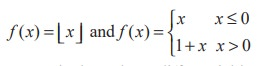Home | | Maths 11th std | Differentiability and Continuity

# Differentiability and Continuity

If a function is continuous at a point, then it is not necessary that the function is differentiable at that point.

Differentiability and Continuity

Illustration 10.3

Test the differentiability of the function f(x) = |x - 2| at x = 2.

Solution

We know that this function is continuous at x = 2.Since the one sided derivatives f ′(2 ) and f ′(2+ ) are not equal, f ′ (2) does not exist. That is, f is not differentiable at x = 2. At all other points, the function is differentiable.

If x0 ≠ 2 is any other point thenThe fact that f ′ (2) does not exist is reflected geometrically in the fact that the curve y = |x - 2| does not have a tangent line at (2, 0). Note that the curve has a sharp edge at (2, 0).

Illustration 10.4

Examine the differentiability of f (x ) = x1/3 at x = 0.

Solution

Let f (x ) = x1/3. Clearly, there is no hole (or break) in the graph of this function and hence it is continuous at all points of its domain.

Let us check whether f ′(0) exists.Therefore, the function is not differentiable at x = 0. From the Fig. 10.19, further we conclude that the tangent line is vertical at x = 0. So f is not differentiable at x = 0.

If a function is continuous at a point, then it is not necessary that the function is differentiable at that point.What can you say about the differentiability of this function at other points?

Illustration 10.5Therefore f ′ (0) does not exist.

Here we observe that the graph of f has a jump at x = 0. That is x = 0 is a jump discontinuity.

The above illustrations and examples can be summarised to have the following conclusions.

A function f is not differentiable at a point x0 belonging to the domain of f if one of the following situations holds:

(i) f has a vertical tangent at x0.

(ii)The graph of f comes to a point at x0 (either a sharp edge or a sharp peak )

(iii) f is discontinuous at x0.

A function fails to be differentiable under the following situations :## We have seen in illustration 10.3 and 10.4, the function f(x) = |x-2| and f(x) = x1/3are respectively continuous at x = 2 and x = 0 but not differentiable there, whereas in Example 10.3 and Illustration 10.5, the functionsare respectively not continuous at any integer x = n and x = 0 respectively and not differentiable too. The above argument can be condensed and encapsuled to state: Discontinuity implies non-differentiability.

### Theorem 10.1 (Differentiability implies continuity)

If f is differentiable at a point x = x0, then f is continuous at x0.

### Proof

Let f(x) be a differentiable function on an interval (a, b) containing the point x0. ThenThis implies, f is continuous at x = x0.

### Derivatives from first principle

The process of finding the derivative of a function using the conditions stated in the definition of derivatives is known as derivatives from first principle.

### EXERCISE 10.1

(1) Find the derivatives of the following functions using first principle.

(i) f (x) = 6 (ii) f(x) = - 4x + 7 (iii) f(x) = - x2 + 2

(2) Find the derivatives from the left and from the right at x = 1 (if they exist) of the following functions. Are the functions differentiable at x = 1?(3) Determine whether the following function is differentiable at the indicated values.

(i) f(x) = x | x | at x = 0

(ii) f(x) = | x 2 - 1 | at x =1

(iii) f(x) = |x| + |x - 1| at x = 0, 1

(iv) f (x ) = sin | x | at x = 0

(4) Show that the following functions are not differentiable at the indicated value of x.(5) The graph of f is shown below. State with reasons that x values (the numbers), at which f is not differentiable.(6) If f(x) = |x + 100| + x2, test whether f ′(−100) exists.

(7) Examine the differentiability of functions in  R by drawing the diagrams.

(i) | sin x |  (ii) | cos x | .Tags : Solved Example Problems, Exercise | Mathematics , 11th Mathematics : UNIT 10 : Differential Calculus: Differentiability and Methods of Differentiation
Study Material, Lecturing Notes, Assignment, Reference, Wiki description explanation, brief detail
11th Mathematics : UNIT 10 : Differential Calculus: Differentiability and Methods of Differentiation : Differentiability and Continuity | Solved Example Problems, Exercise | Mathematics